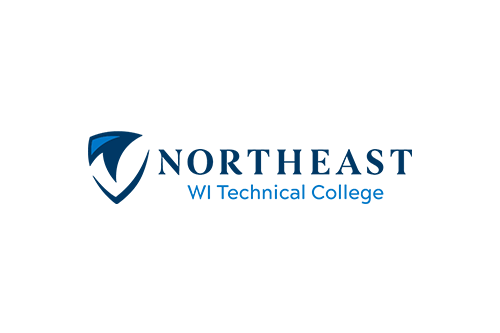Credits: 2
Course Number: 10-804-312

## Course Description

10-804-312 MATH-ALGEBRA/TRADES ...signed numbers, order of operations, scientific notation, metric units/measurement, calculator operations, algebra, introductory trigonometry, Pythagorean Theorem, solving right triangles. (Prerequisite: Next Gen Arith score greater/equal to 237 AND Rdg score greater/equal to 237; OR TABE A 9/10 Math greater/equal to 9.2 AND Rdg greater/equal to 8.3; OR TABE 11/12 Math greater/equal to 602 AND Rdg greater/equal to 567; OR ACT Math score greater/equal to 14 AND ACT Rdg score greater/equal to 15; OR prep courses-contact academic advisor 920-498-5444)

• Summer

## What will I learn?

1. Multiply and divide values in scientific and engineering notations
2. Convert units of measure using dimensional analysis
3. Evaluate formulas
4. Solve linear and literal equations
5. Solve for the components of a right triangle using the trigonometric functions
Filter by

## No classes available

Registration is not currently available for this course. Learn more about registration dates and how to register.

Return to search